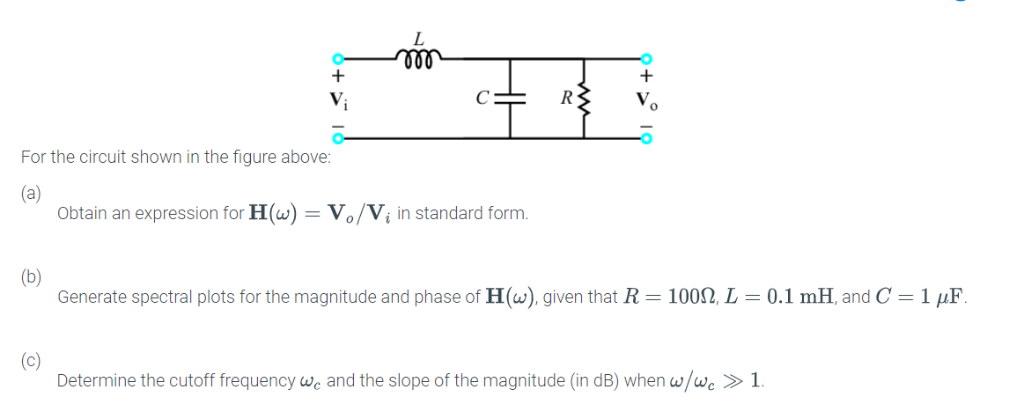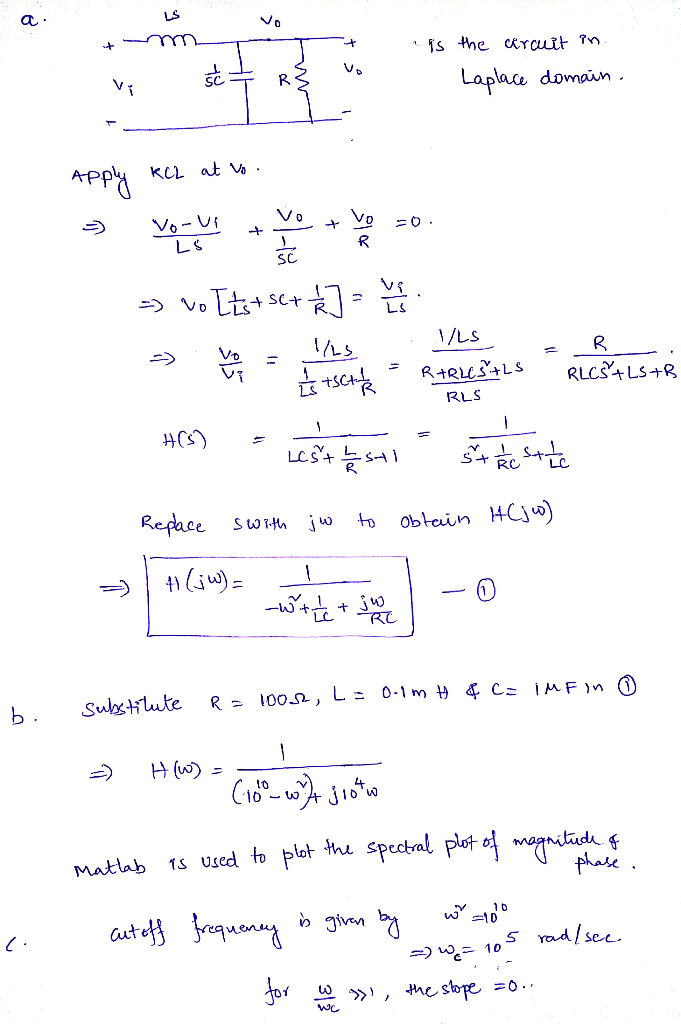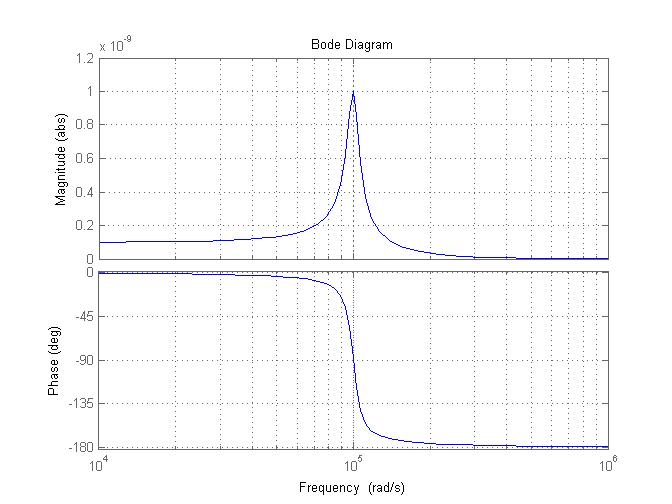# For the circuit shown in the figure above: Obtain an expression for H() Vo/Vi in standard form Generate spectral plots for the magnitude and phase of H (w), given that R-100Ω, L-0.1 mH, and C-1 μF De...For the circuit shown in the figure above: Obtain an expression for H() Vo/Vi in standard form Generate spectral plots for the magnitude and phase of H (w), given that R-100Ω, L-0.1 mH, and C-1 μF Determine the cutoff frequency we and the slope of the magnitude (in dB) when wwc >1MATLAB code is given below in bold letters.

r=100;l=0.1e-3;c=1e-6;
h=tf(1,[1 1/(r*c) 1/(l*c)])
figure;bode(h);grid on;

The magnitude and phase responses are plotted below.##### Add Answer of: For the circuit shown in the figure above: Obtain an expression for H() Vo/Vi in standard form Generate spectral plots for the magnitude and phase of H (w), given that R-100Ω, L-0.1 mH, and C-1 μF De...
More Homework Help Questions Additional questions in this topic.